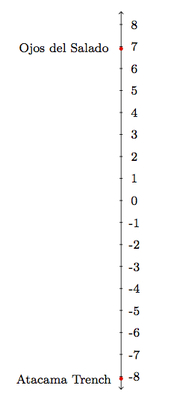# Differences of Integers

Alignments to Content Standards: 7.NS.A.1.c 7.NS.A.1.b 7.NS.A.1

Ojos del Salado is the highest mountain in Chile, with a peak at about 6900 meters above sea level. The Atacama Trench, just off the coast of Peru and Chile, is about 8100 meters below sea level (at its lowest point).

1. What is the difference in elevations between Mount Ojos del Salado and the Atacama Trench?
2. Is the elevation halfway between the peak of Mount Ojos del Salado and the Atacama Trench above sea level or below sea level? Explain without calculating the exact value.
3. What elevation is halfway between the peak of Mount Ojos del Salado and the Atacama Trench?

## IM Commentary

The goal of this task is to subtract integers in a real world context. It will be very helpful for students to use number lines for this task. In the solution they are drawn vertically to match the context of elevation but accurately labeled horizontal number lines are also appropriate. More information about the geographic features mentioned in the task statement can be found at

## Solution

1. Below is a number line showing the elevation of these two locations. Each unit on the number line represents 1000 feet of elevation:The number line indicates that to find the difference in elevation from the peak of Ojos del Salado to the bottom of the Atacama Trench, we need to add the elevation of Ojos del Salado above sea level to the depth of the Atacama Trench below sea level. In equations, we have \begin{align} 6900 - (-8100) &= 6900 + 8100 \\ &= 15000.\end{align} There is a difference of 15,000 meters between the altitudes of these two locations on the earth. In this equation, 8100 = -(-8100): subtracting -8100 is the same as adding 8100.

2. Since 8100 $\gt$ 6900, the depth of Atacama Trench is greater than the height of Ojos del Salado. If these quantities were the same, then the midpoint between them would be at sea level. Since the depth of Atacama Trench is greater, this means that the midpoint between these two quantities is negative or below sea level.
3. One way to find the elevation halfway between the mountain peak and trench floor is to divide the 15,000 foot difference between these by 2: 15,000 $\div$ 2 = 7500. This means that the point midway between them will be 7500 feet below the mountain peak and 7500 feet above the ocean floor: 6900 - 7500 = -600 and similarly -8100 + 7500 = -600. So the elevation halfway between the mountain peak and trench floor is at -600 feet.

A second method uses an idea from statistics. The midpoint between 6900 and -8100 the mean of the two numbers: \begin{align} \frac{6900 + (-8100)}{2} &= \frac{6900-8100}{2}\\ &= \frac{-1200}{2} \\ &= -600.\end{align} Halfway between the peak of Ojos del Salado and the bottom of the Atacama Trench is 600 meters below sea level. This is shown in the number line below: